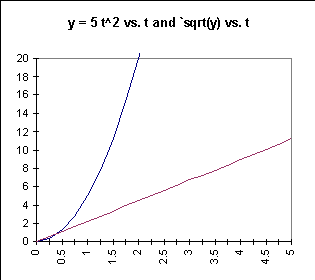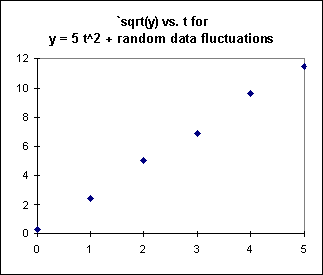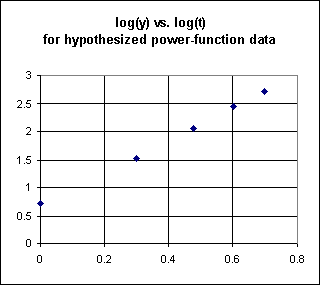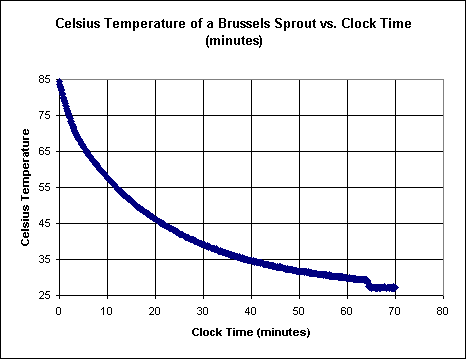## Linearizing Data and Curve Fitting with Logarithms

#### Introduction

We often encounter situations in which we expect that our data is of a certain type. For example we might expect a proportionality of the form y = a t^p, for some power p. Or we might have reason to expect that our data is exponential, of form y = a b^t. These forms involve exponents, and are most easily analyzed with the help of logarithms.

In many cases our simplest option for analysis will be to 'linearize' our data, to transform it into data whose graph is linear, in order to do a linear regression fit of the form y = mx + b. After obtaining this function we then transform it back into its original form to obtain our model.

We begin with a function that doesn't require logarithms. The function we will use will be of the form y = A t^2. We are aware that the squaring function is 'undone' by its inverse function, the square root function. We will therefore transform square-function data into linear data by taking the square root. Once we have a linear model for the transformed data we will return to the original form by squaring this function.

We will then see how to linearize exponential functions, power functions and logarithmic functions.

#### Exercises 1-3

1. Make a table for y = 2 t^2 vs. t, for t = 0 to 3.

Using the y and t values from this table, create a second table of `sqrt(y) vs. t.

Perform a sequence analysis on the `sqrt(y) column, showing that the first difference of the `sqrt(y) sequence is constant and nonzero.

Determine the values of m and b for the linear function `sqrt(y) = mt + b that models your `sqrt(y) vs. t table. You may graph this equation and measure slope and y intercept if necessary, but the table should give you this information without requiring a graph. DERIVE or another curve fitting option would also give you the desired result, but this should really be unnecessary in this situation.

Square this linear function. You should get the original function y = 2 t^2.

2. Repeat the process of the first exercise for the exponential function y = 7 (3 ^ t):

Make a table of y vs. t for t = 0 to 3.

Using the y and t values from this table create a second table of log(y) vs. t.

Perform a sequence analysis on the log(y) column to show that this table is modeled by a linear function.

Determine the values of m and b for the linear function log(y) = mt + b that models this table. Once again the table should give you the necessary information, but you may if necessary use a graph. DERIVE should be unnecessary here.

Write the equation 10 ^ log(y) = 10 ^ (mt + b), using the values of m and b obtained in the previous step.

Use the laws of exponents and logarithms to simplify this equation into the form y = a b^t.

Your result should match the original function.

3. For the function y = 3 (t ^ 1.5), follow the following process:

Make a table of y vs. t for t = 1 to 4.

Using the y and t values from this table create a second table of log(y) vs. log(t).

Show that this table is linear in nature by calculating the rates of change between consecutive data points. All the rates should be identical.

Find the values of m and b for the linear function log(y) = m log(t) + b

Write the equation 10 ^ log (y) = 10 ^ (m log(t) + b).

Use the laws of exponents and logarithms to simplify this equation into the form y = a t ^ p.

Your result should match the original function.

#### If your data contained errors or uncertainties, as real-world data almost always does, then the process would have discovered an approximation to the original function. The approximation would tend to be better if the errors or uncertainties were smaller.

The next several sections work out the details of the process used in these exercises.

#### Linearizing y = A t^2 with the Square Root Function

Example: The function y = 5 t^2

A table of the function y = 5 t^2 is shown below:

 t y = 5 t^2 0 0 1 5 2 20 3 45 4 80 5 125

The corresponding table of `sqrt(y) vs. t is found by replacing all y values by `sqrt(y):

 t `sqrt(y) 0 0 1 2.24 2 4.47 3 6.70 4 8.94 5 11.18

A graph of `sqrt(y) vs. t yields a straight line, as shown on the graph of y = 5t^2 vs. t and `sqrt(y) vs. t below:Sequence analysis tells us that the function is linear: We find something striking when we do a sequence analysis of the numbers in the `sqrt(y) column. The sequence of `sqrt(y) values is

0 2.24   4.47 6.70    8.94 ... .

This sequence has a common difference of approximately 2.24.

It follows from the difference analysis that `sqrt(y) is a linear function of t, with slope 2.24. Since `sqrt(y) = 0 when t = 0, we have `sqrt(y) = 2.24 t + 0 = 2.24 t.

It is too hard to see why we obtain a linear function from this process. Since for t >= 0,

`sqrt(y) = `sqrt(5 t^2)

= `sqrt(5) * `sqrt(t^2)

= `sqrt(5) * t

= 2.24 t

with the number a representing `sqrt(5) to 3 significant figures, we see why the linear function `sqrt(y) = 2.24 t gave us a good model of the table.

Terminology: Data transformation and linearization: In the above example we have transformed the equation y = 5 t^2, which doesn't have a linear graph, into the equation z = `sqrt(y) = 2.24 t^2, which does have a linear graph z vs. t. We therefore say that we have linearized the function.

Application of the process of linearization to a real-world data set: Suppose now that we had a set of observations of a quantity y vs. time t. Imagine that in reality the quantity y was related to t by the equation y = 5 t^2, but that our observations were not completely accurate. Instead of the values on the previous table we might obtain the following values:

 t y = ??? 0 0.14 1 5.66 2 23.2 3 52.0 4 87.2 5 135.5

These values are close to but not identical with the values on our original table. If we take the square root of the y values we obtain the following table:

 t `sqrt(y) 0 0.37 1 2.38 2 4.82 3 7.21 4 9.34 5 11.6

This table represents our attempt to linearize the data.

A graph of these `sqrt(y) vs. t data points, shown below, doesn't yield a perfect straight line, but the result is nearly linear. So our attempt to linearize the data was pretty successful.Interpreting the y = mx + b form given by the curve fitting utility

When we do a curve fit to the data, DERIVE or whatever utility we use to obtain the fit will very likely give us our result in the form y = mt + b. However, we know that the form should be `sqrt(y) = mt + b, since our data was `sqrt(y) vs. t. This is because the curve-fitting utility doesn't have a clue that we are looking for a `sqrt(y) function. All the program knows is that we want a linear fit to some data set. It doesn't make interpretations, it just does the number crunching. And when it crutches a data set it gives us a 'y =' message. It is up to us to ignore the 'y=' notation and interpret the result as '`sqrt(y) ='.

So when the computer tells us, for example, that the linear function is y = 2.31 t + .09, we must think about what our data meant. Our data was `sqrt(y) vs. t, not y vs. t. So we interpret the y = 2.31 t + .09 function as really meaning `sqrt(y) = 2.31 t + .09.

A linear y = mt + b curve fit to this data will ideally give us y = a t (really meaning `sqrt(y) = a t), but the inaccuracy of our observations will almost certainly result in a different function.

For example the fit might give us the linear function `sqrt(y) = mt+b = 2.21 t + .03, or 2.26 t - .05, or 2.05 t + .31, etc.. The more accurate our data the closer the coefficient m will be to 2.24, and the closer the y-intercept b will be to 0.

Of the three example functions given here, 2.05 t + .31 is the furthest from the ideal model, with both m and b being further from the ideal values m = 2.24 and b = 0 than either of the other two models.

Using the `sqrt(y) = mt + b function to get the original y vs. t function

We can use the best-fit function for our linearized data to figure out the original function. Suppose for example that DERIVE gave us a best-fit function y = 2.27 t + .05 for the data of the above table. We would begin by interpreting this as `sqrt(y) = 2.27 t + .05.

We wish to obtain the y vs. t function for the original data from this linearized function. We do this by simply solving for y the equation for the linearized data:

 `sqrt(y) = 2.27 t + .05 this is the equation of the linearized `sqrt(y) vs. t data (`sqrt(y))^2 = (2.27 t + .05) ^ 2 we square both sides to obtain y (using inverse function) y = 5.15 t^2 + .227 t + .0025 we expand the squares y = 5.15 t^2 (approx.) we approximate by omitting the relatively small quantity .227 + .0025

The above process is pretty obvious. But we need to note two things:

Data was linearized with the square root function; the linear function was 'unlinearized' with the inverse, or squaring, function:  When we squared both sides of the equation we used the squaring function, which is inverse to the square root function used to linearize the data. So we linearized the data with one function (the square root function) and then we 'unlinearized' it with the inverse function (the square root function).

We neglected small quantities that didn't fit with our desired model:  When we neglected the .227 t + .0025, we noted that compared to 5.15 t^2 these quantities were small. For values of t which are not close to 0 this is so.

The neglecting of small quantities was justified by the fact that our data was imperfect:  Neglecting these quantities would be justified by our expectation that the desired function has the form y = A t^2 rather than y = a t^2 + bt + c. For example if we were looking at areas of geometrically similar objects we would expect the proportionality y = A t^2 rather than the quadratic y = a t^2 + bt + c. We would therefore treat the small quantity .227 t + .0025 as an accidental result of the inaccuracy of our data, and not as an important aspect of the behavior of the system.

So from the y = 2.27 t + .05 linear fit (really `sqrt(y) = 2.27 t + .05) we obtain from our data the function y = 5.15 t^2.

#### Exercises 4-7

4.  Make a table for y = 9 t^2. Then make a table for `sqrt(y) vs. t. Sketch graphs of both tables.

What linear function `sqrt(y) = mt + b fits your second table? (Use sequence analysis as above. If necessary you could do a linear fit to your table).

Take the `sqrt of both sides of the equation y = 9 t^2 and simplify the right-hand side. What do you get?

5.  What do you get when it take the square root of both sides of the equation y = a t^2?

What would you expect the slope of the graph of `sqrt(y) vs. t to be?

6.  Make a table for y = 4 t^2 + 3. Then make a table for `sqrt(y) vs. t. Sketch a graph of the second table. Does the graph appear to give you a straight line? Does sequence analysis give you a straight line?

Take the square root of both sides of the equation.

Would you expect a graph of sqrt(4 t^2 + 3) to be of the form mx + b? Can you justify your answer?

7.  Using the table of simulated real-world data above, we hypothesized the fit y = 2.27 x + .05 (actually `sqrt(y) = 2.27 x + .05). Do your own linear fit dist this data and follow the above process to obtain the corresponding y = A t^2 function. Compare your result to the 'ideal' y = 5 t^2 function.

#### Generalizing the process of curve fitting by linearization

The process used above followed a well-defined sequence of steps:

1. Hypothesize a function model (y = A t^2 in the above example, with basic function y = t^2)

2. Transform using an appropriate inverse function to linearize the data (use the `sqrt function in the above example)

3. Obtain a linear fit to the data (e.g., using fit([t,mt+b] ... in DERIVE)

4. If necessary, interpret the 'y =' form of the linear fit (use '`sqrt(y) =' in above)

5. Solve the resulting equation by applying the inverse function to both sides (square both sides in above)

6. If appropriate, neglect small terms (neglect .227t + .0025 in above)

7. Compare your final model with the original data (not done above)

We can generalize this procedure to include a broader class of functions as follows:

 1.   Hypothesize the basic function to which our data is proportional (e.g., exponential, power-function, logarithmic) 2.   Transform y and/or t using appropriate inverse function(s) to linearize the data 3.   Obtain a linear fit to the data, probably in y = mx + b form 4.   Reinterpret the linear function, replacing y and x with appropriate expressions 5.   Solve the resulting equation for the dependent variable 6.   If appropriate neglect small terms 7.   Compare the final model with original data.

Next we will apply this procedure to a variety of function models, including exponential functions y = a (2^(kt)), power functions y = a t^p, and logarithmic functions y = a log(t) + c.

Application to the exponential function y = A (2^(kx))

If y = a (2^(kt)), then if we apply the two basic rules of logarithms log(ab) = log(a) + log(b) and log(a^b) = b log(a) we obtain

log(y) = log(a (2^(kt))

= log(a) + log(2^(kt))

= log(a) + kt log(2).

This can be rearranged to give us log(y) = k log(2) t + log(a). This is a linear function of the form mx + b, with m = k log(2) and b = log(a) and x = t. It follows that if we graph log(y) vs. t, we should get a straight line with slope k log(2) and vertical intercept log(a).

For example if y = 3 (2^(.4 t)) then

log(y) = log(3) + .4 log(2) t = .477 + .4(.301) t = .477 + .120 t.

If we graph this expression vs. t we will get a straight line with slope .120 and y-intercept .477.

If we make a table of y = 3 (2^(.4 t)) vs. t, we will obtain approximate values

 x y 0 3.00 1 3.96 2 5.22 3 6.89 4 9.09 5 12.0

If we linearize this 'data' by replacing y with log(y) we will obtain the table

 x log(y) 0 0.48 1 0.60 2 0.72 3 0.84 4 0.96 5 1.08

A DERIVE linear fit to this table would give us y = .120 t + .477.

We interpret this function as log(y) = .120 t + .477.

We then solve for y, using the inverse function y = 10^x to the y = log(x) function with which we transformed the data:

y = 10 ^ (log(y)) = 10 ^ (.120 t + .477)

= 10^.477 * 10^(.120 t)

= 3 * 1.32 ^ t.

Since 2 ^ .4 = .132, 2 ^ (.4 t) = (2^.4) ^ t = 1.32 ^ t, and we see that the function y = 3 * 1.32 ^ t is identical to the function we started with.

#### Exercises 8-9

8.  If our real world data set was slightly different from the one given above, due to uncertainties in measurement or deviations from ideal behavior, our table might look like this:

 x y 0 2.9 1 3.8 2 5.4 3 6.9 4 9.2 5 11.8

These data will result in a slightly different model than the one obtained above. Transform the data using the logarithms of the y column, then follow the rest of the procedure to obtain the final y vs. t model. Compare with the model y = 3 * 1.32 ^ t obtained for the original data set.

Note how you have followed the first 6 steps of the 7-step procedure outlined above.

9.  Assuming that the data is exponential (this is the assumption you will make for step 1 of the 7-step procedure), follow the entire 7-step procedure for the data set

 x y 0 0.42 1 0.29 2 0.21 3 0.15 4 0.10 5 0..07

For the seventh step determine the average deviation of your function from the given data.

Application to the power function y = a t ^ p

In our previous efforts to fit power functions to data, we knew what power we were looking for. This made the process pretty simple, since we had only one parameter to determine.

However we often wish to fit a power function to a data set without knowing the power involved. We explore here a technique for fitting a power function y = a t ^ p with the two parameters a and p to a data set.

If we do a logarithmic transformation of y = a t ^ p, we get

log(y) = log(a t^p )

= log(a) + log(t^p)

= log(a) + p log(t).

We note that t remains bound up in the log function, unlike the case for the exponential function of the preceding example.

We can get around this problem if we simply let x = log(t). We then get

log(y) = log(a) + p log(t)

= p log(t) + log(a)

= p x + log(a).

This is of the form mx + b with m = p and b = log(a).

We will therefore get a straight line if we graph (p x + log(a) ) vs. x. The slope of the line will be the power p, and the y intercept will be the logarithm of the confusion coefficient a.

Of course x = log(t), so when we create our table prior to graphing we will need to set up the columns log(y) = p x + log(a) vs. x = log(t).

Example: The data set below was created by adding random 'errors' to the function y = 4 x^3:

 x y 1 5.29 2 32.74 3 116.7 4 272.5 5 506.0

Assuming that we know we are looking for a power function, we would see from the above discussion that we need to create a table of log(y) vs. log(t):

 log(t) log(y) 0 0.72 0.30 1.57 0.47 2.07 0.60 2.44 0.69 2.72

A graph of log(y) vs. log(x) would lie nearly along a straight line.We can estimate the linear regression line from this data. We might obtain something like y = 2.9 x + .7. Then if we are paying attention we will remember that y on this graph is really log(y), and x is really log(t). So we would write

log(y) = 2.9 log(t) + .7.

Having obtained this relationship from the transformed data we can proceed to reverse the transformation. To do so we simply solve for y. Recalling that the 10^x function is inverse to the log(x) function we use the 10^x function to reverse the transformation:

10 ^ (log(y)) = 10^(2.9 log(t) + .7)

Using the laws of exponents we obtain

y = 10^(2.9 log(t) ) * 10 ^ .7

y = (10 ^ log(t) ) ^ 2.9 * 10^.7

y = t ^ 2.9 * 5.01

y = 5.01 t ^ 2.9.

Recall that the original data was generated by adding random errors to the function y = 4 t ^ 3. The added errors resulted in a coefficient of 5.01 rather than 4, and a power of 2.9 rather than 3. It looks like we got the power pretty close but the coefficient was off by about 25%; however this is not due to an error in our procedure, but to the errors inserted into our original data. The data set was simply not accurate enough to give us a precise result.

#### Exercise 10

10.  Use a curve fitting utility to obtain a better regression line for the above transformed data set and determine whether you end up with a model significantly closer to the 'ideal' y = 4 t^3 function.

Assuming that the data set

 t y 1 2.42 1.5 6.78 2 19.1 2.5 22.2 3 45.8

is generated by a power function, use the appropriate transformations with the 7-step model to obtain a power function model for the data.

11.  For each of the following data sets determine whether the log(y) vs. t or the log(y) vs. log(t) transformation works better, and for the better transformation find the linear model of the transformed data.   Then use the inverse transformation to obtain the y vs. t model.  Determine the average discrepancy between the function and your data.

 t y .5 .7 1 .97 1.5 1.21 2 1.43 2.5 1.56

 t y 2 2.3 4 5.1 6 11.5 8 25

12.  For the graph below, which shows the temperature of a Brussels sprout (mass approx. 25 grams) left in a constant-temperature room,  first determine the asymptote to which the temperature seems to approach (note:  at the end of the experiment the temperature probe was removed from the sprout and dried off), then using four equally spaced graph points determine whether the temperature difference Td = Ts - Tr (Td is temp. diff., Ts is temperature of the sprout and Tr is the asymptotic temperature) appears to be proportional to the rate of temperature change.  Then determine the proportionality constant of this proportionality.

Using ten equally spaced representative points on the graph, linearize Td vs. clock time t, determine the best-fit line for this lienarized data, then use the appropriate inverse function to 'unlinearize' the data.  Determine the quality of the resulting curvefit.